We’re moving the GATK website, docs and forum to a new platform. Read the full story and breakdown of key changes on this blog.
Notice:
If you happen to see a question you know the answer to, please do chime in and help your fellow community members. We encourage our fourm members to be more involved, jump in and help out your fellow researchers with their questions. GATK forum is a community forum and helping each other with using GATK tools and research is the cornerstone of our success as a genomics research community.We appreciate your help!

#### Test-drive the GATK tools and Best Practices pipelines on Terra

Check out this blog post to learn how you can get started with GATK and try out the pipelines in preconfigured workspaces (with a user-friendly interface!) without having to install anything.

# (Notebook) Correlate gCNV callset metrics and annotations

edited April 1

This notebook examines gCNV callset annotations using data from Tutorial#11684. The tutorial focuses its illustrations on small data from a single sample NA19017. This notebook analysis uses larger data, namely gCNV results from the tutorial's 24-sample cohort. The notebook plots annotations from gCNV results that used default model fitting parameters and from gCNV results that used sensitive model fitting parameters. Finally, the notebook collects metrics for each sample's gCNV callset and generates a clustered heatmap from the metrics.

The sections of Notebook#11686 are as follows.

Download an HTML version and the `.ipynb` format notebook here.

### Install and import Python packages

```# Prerequisites include Python 3, Jupyter and GATK4.
# Notebook results use v3.7.1, v4.4.0 and v4.1.0.0, respectively, and assumes a Unix environment.
! pip install numpy pandas matplotlib seaborn
```
```import glob, os
import numpy as np
import pandas as pd
import matplotlib.pyplot as plt
import seaborn as sns; sns.set()
```

# 1. Annotation profiles for default parameter results

### 1.1 Convert VCF annotations of interest to a dataframe table

gCNV segmented VCF results were previously converted to table format using the following command.

```gatk VariantsToTable \
-V genotyped-segments-NA19017.alt_bwamem_GRCh38DH.20150917.LWK.high_coverage.vcf.gz \
-O annotations_NA19017.table \
-F CHROM -F POS -F END -GF CN -GF NP -GF QA -GF QS -GF QSE -GF QSS
```
```# Navigate to directory with the tutorial data and list tables.
! ls *.table
```
```# Generate a dataframe of the tablular data that includes all samples and is subset to chr20.
gcnvAll = [];
for file in glob.glob("annotations_*.table"):
aColumnName = gcnvLoop.columns
sampleNameString = aColumnName[0:-3]
gcnvLoop.columns = ['CHROM', 'POS', 'END', 'CN', 'NP', 'QA', 'QS', 'QSE', 'QSS']
gcnvLoop.insert(loc=0, column='sample', value=sampleNameString)
gcnvLoop20=pd.DataFrame(gcnvLoop.loc[gcnvLoop['CHROM'] == 'chr20'])
gcnvAll.append(gcnvLoop20[['sample', 'CN', 'NP', 'QA', 'QS', 'QSE', 'QSS']])

gcnvAll = pd.concat(gcnvAll, axis=0, sort=False)
gcnvAll
```
```# Define subsets of dataframe by event type

# Normal diploid
a = gcnvAll.loc[gcnvAll['CN'] == 2]

# Deletions
b = gcnvAll.loc[gcnvAll['CN'] < 2]

# Amplifications
c = gcnvAll.loc[gcnvAll['CN'] > 2]

# Deletions and amplifications
d = b.append(c)
```

### 1.2 Plot histograms of each event type

```# Plot the distributions as histograms.
fig, axs = plt.subplots(1,3, figsize=(18,6))
sns.distplot(a['NP'], ax=axs, kde=False)
sns.distplot(b['NP'], ax=axs, kde=False)
sns.distplot(c['NP'], ax=axs, kde=False)

axs.set_title('Normal', fontsize = 18)
axs.set_title('Deletions', fontsize = 18)
axs.set_title('Amplifications', fontsize = 18)
plt.suptitle('Histograms of gCNV event sizes', fontsize = 18)
axs.set_ylabel('log counts', fontsize=18)
axs.set_xlabel('size (kb)', fontsize = 18)

axs.set_yscale('log')
axs.set_yscale('log')
axs.set_yscale('log')
axs.set_xlim(0,15000)
axs.set_ylim(0.8,2000)
axs.set_xlim(0,80)
axs.set_ylim(0.8,2000)
axs.set_xlim(0,80)
axs.set_ylim(0.8,2000)
```
```(0.8, 2000)
```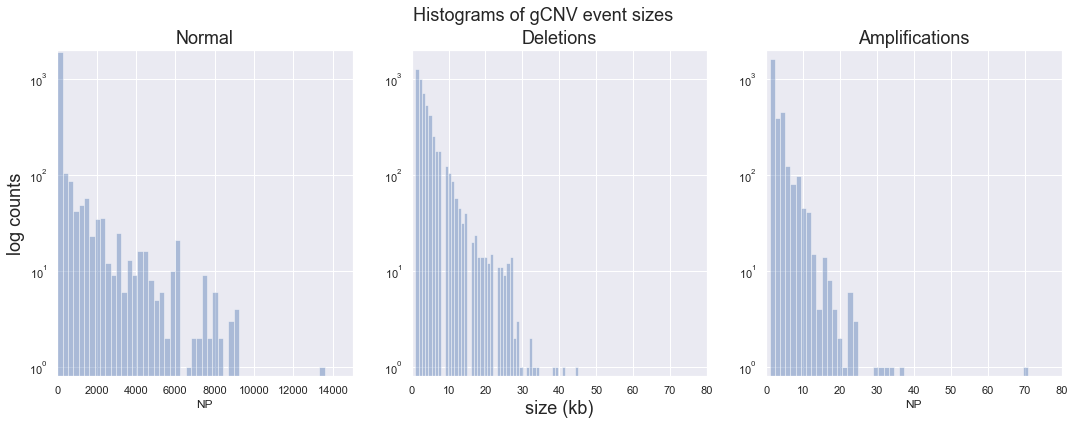### 1.3 Plot quality scores (QA and QS) and size (NP)

```# Plot quality scores QA and QS and size (NP) against each other.
# For the data, each NP (number of points) corresponds to a 1kb bin.
##FORMAT=<ID=CN,Number=1,Type=Integer,Description="Segment most-likely copy-number call">
##FORMAT=<ID=NP,Number=1,Type=Integer,Description="Number of points (i.e. targets or bins) in the segment">
##FORMAT=<ID=QA,Number=1,Type=Integer,Description="Complementary Phred-scaled probability that all points (i.e. targets or bins) in the segment agree with the segment copy-number call">
##FORMAT=<ID=QS,Number=1,Type=Integer,Description="Complementary Phred-scaled probability that at least one point (i.e. target or bin) in the segment agrees with the segment copy-number call">

fig, axs = plt.subplots(2,2, figsize=(18,12))
sns.scatterplot(x=d['NP'], y=d['QS'], hue=d['CN'], size=d['NP'], data=d, ax=axs[0,0], palette="RdBu")
sns.scatterplot(x=d['QA'], y=d['QS'], hue=d['CN'], size=d['NP'], data=d, ax=axs[0,1], palette="RdBu")
sns.scatterplot(x=d['NP'], y=d['QS']/d['NP'], hue=d['CN'], size=d['NP'], data=d, ax=axs[1,0], palette="RdBu")
sns.scatterplot(x=d['QA'], y=d['QS']/d['NP'], hue=d['CN'], size=d['NP'], data=d, ax=axs[1,1], palette="RdBu")

plt.suptitle('gCNV annotation plots (deletions & amplifications)', fontsize = 18)
axs[1,0].set_ylabel('QS/NP', fontsize=12)
axs[1,1].set_ylabel('QS/NP', fontsize=12)
```
```Text(0, 0.5, 'QS/NP')
```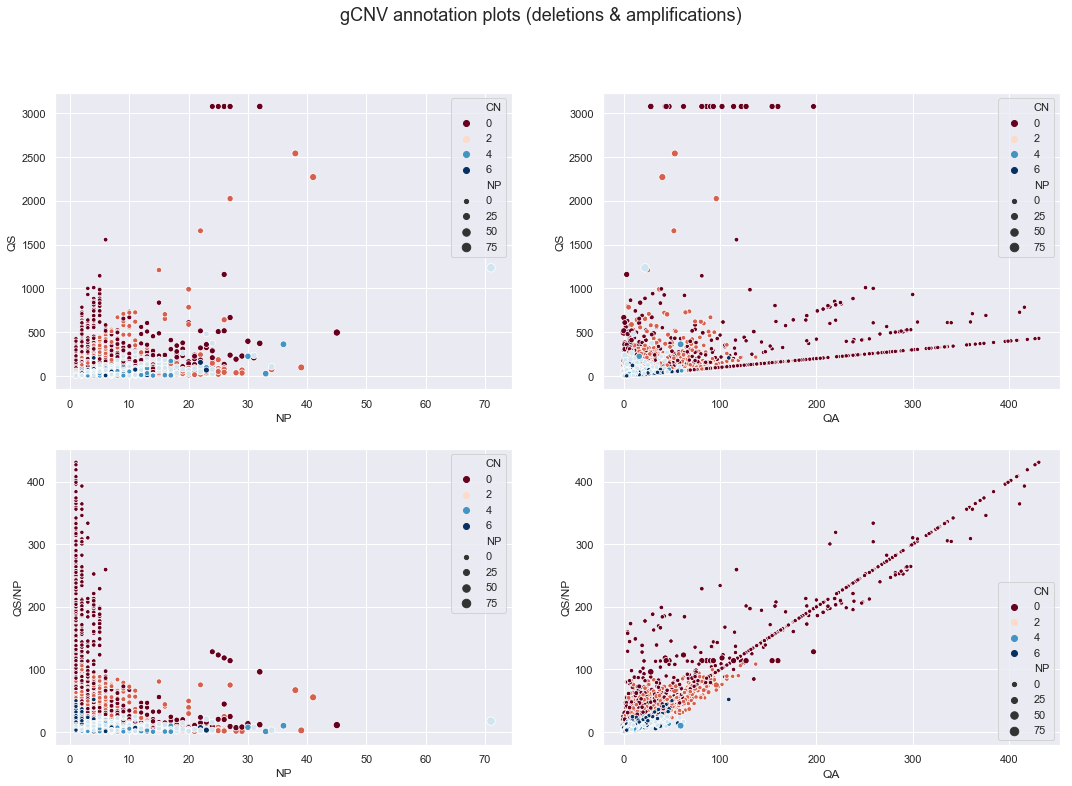A number of trends are apparant, especially after normalizing quality scores by NP (bottom two plots).

• Copy number states cluster. Deletions appear to have higher quality than insertions.
• CN0 and CN4+ events are more confident than CN1 and CN3 events, respectively. That is, the closer the copy number state is to normal--here diploid--the harder it is to detect events.
• QS appears capped at 3077 and also has a lower bound that increases with QA.

The next set of plots separate deletion and amplification event types.

```# Segment by event type and plot
fig, axs = plt.subplots(2,2, figsize=(18,12))

sns.scatterplot(x=b['NP'], y=b['QS']/b['NP'], hue=b['CN'], size=b['NP'], data=b, ax=axs[0,0], palette="mako")
sns.scatterplot(x=c['NP'], y=c['QS']/c['NP'], hue=c['CN'], size=c['NP'], data=c, ax=axs[0,1], palette="mako")
sns.scatterplot(x=b['QA'], y=b['QS']/b['NP'], hue=b['CN'], size=b['NP'], data=b, ax=axs[1,0], palette="mako")
sns.scatterplot(x=c['QA'], y=c['QS']/c['NP'], hue=c['CN'], size=c['NP'], data=c, ax=axs[1,1], palette="mako")

plt.suptitle('gCNV annotation plots', fontsize = 18)
axs[0,0].set_title('Deletions', fontsize=18)
axs[0,1].set_title('Amplifications', fontsize=18)
axs[0,0].set_ylabel('QS/NP', fontsize=12)
axs[0,1].set_ylabel('QS/NP', fontsize=12)
axs[1,0].set_ylabel('QS/NP', fontsize=12)
axs[1,1].set_ylabel('QS/NP', fontsize=12)
```
```Text(0, 0.5, 'QS/NP')
```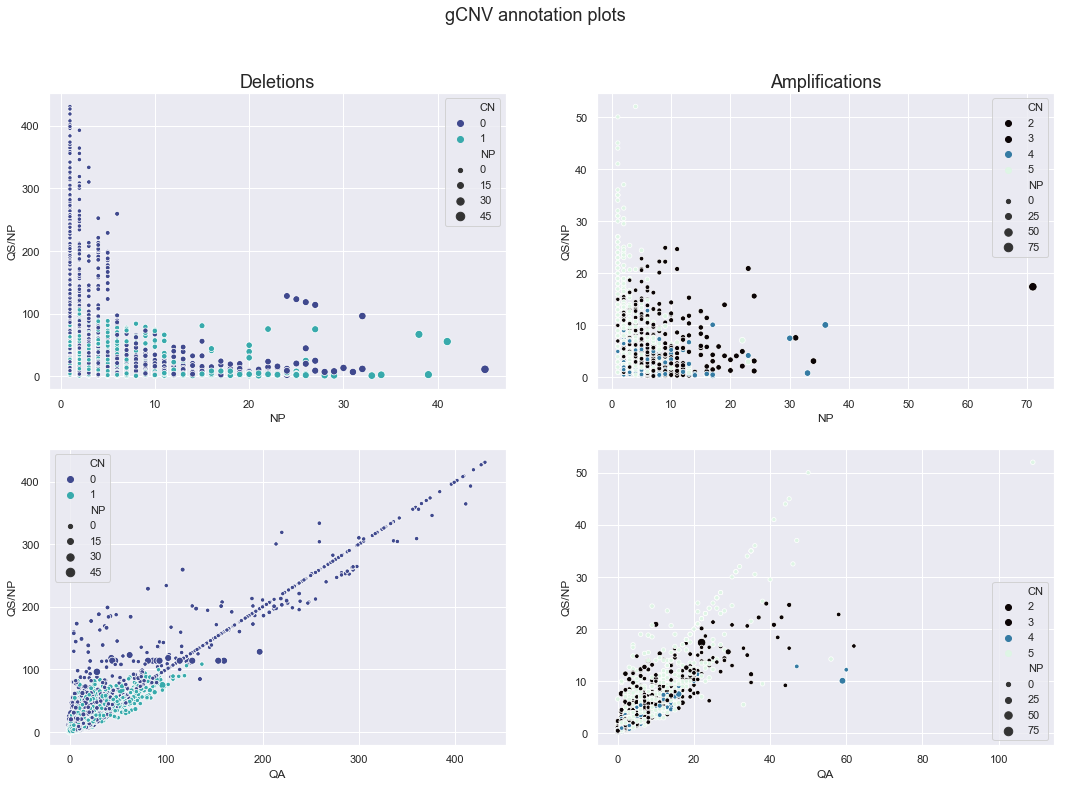# 2. Annotation profiles for sensitive parameter results

2.1 Convert VCF annotations of interest to a dataframe table

```# Navigate to directory with the tutorial data and list tables.
! ls *.table
```
```# Generate a dataframe of the tablular data that includes all samples and is subset to chr20.
gcnvAllSensitive = [];
for file in glob.glob("annotations_*.table"):
aColumnName = gcnvLoop.columns
sampleNameString = aColumnName[0:-3]
gcnvLoop.columns = ['CHROM', 'POS', 'END', 'CN', 'NP', 'QA', 'QS', 'QSE', 'QSS']
gcnvLoop.insert(loc=0, column='sample', value=sampleNameString)
gcnvLoop20=pd.DataFrame(gcnvLoop.loc[gcnvLoop['CHROM'] == 'chr20'])
gcnvAllSensitive.append(gcnvLoop20[['sample', 'CN', 'NP', 'QA', 'QS', 'QSE', 'QSS']])

gcnvAllSensitive = pd.concat(gcnvAllSensitive, axis=0, sort=False)
gcnvAllSensitive
```

The sensitive parameters give ~54K rows, which is roughly 5x more than the default parameter's ~11K rows.

```# Define subsets of dataframe by event type

# Normal diploid
a = gcnvAllSensitive.loc[gcnvAllSensitive['CN'] == 2]

# Deletions
b = gcnvAllSensitive.loc[gcnvAllSensitive['CN'] < 2]

# Amplifications
c = gcnvAllSensitive.loc[gcnvAllSensitive['CN'] > 2]

# Deletions and amplifications
d = b.append(c)
```

### 2.2 Plot histograms of each event type

```# Plot the distributions as histograms.
fig, axs = plt.subplots(1,3, figsize=(18,6))
sns.distplot(a['NP'], ax=axs, kde=False)
sns.distplot(b['NP'], ax=axs, kde=False)
sns.distplot(c['NP'], ax=axs, kde=False)

axs.set_title('Normal', fontsize = 18)
axs.set_title('Deletions', fontsize = 18)
axs.set_title('Amplifications', fontsize = 18)
plt.suptitle('Histograms of gCNV event sizes', fontsize = 18)
axs.set_ylabel('log counts', fontsize=18)
axs.set_xlabel('size (kb)', fontsize = 18)

axs.set_yscale('log')
axs.set_yscale('log')
axs.set_yscale('log')
axs.set_xlim(0,80)
axs.set_xlim(0,80)
```
```(0, 80)
```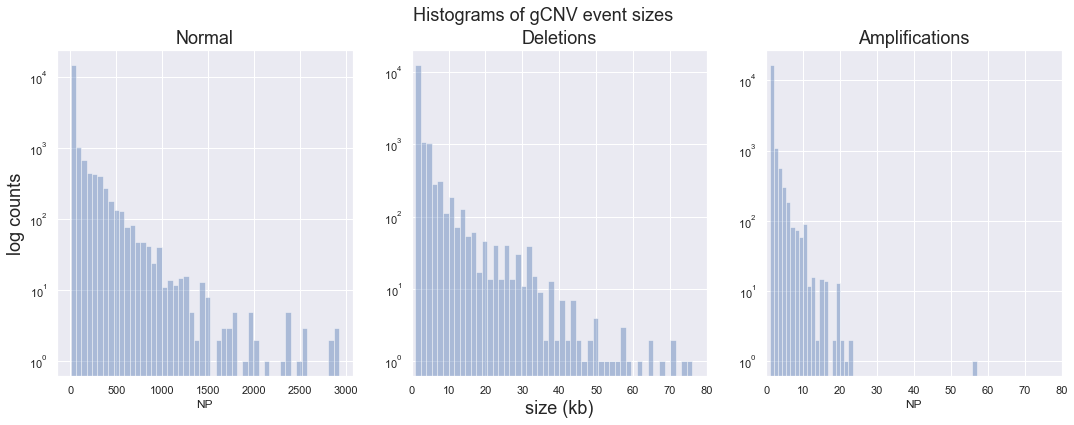### 2.3 Plot quality scores (QA and QS) and size (NP)

```# Plot quality scores QA and QS and size (NP) against each other.
# For the data, each NP (number of points) corresponds to a 1kb bin.
##FORMAT=<ID=CN,Number=1,Type=Integer,Description="Segment most-likely copy-number call">
##FORMAT=<ID=NP,Number=1,Type=Integer,Description="Number of points (i.e. targets or bins) in the segment">
##FORMAT=<ID=QA,Number=1,Type=Integer,Description="Complementary Phred-scaled probability that all points (i.e. targets or bins) in the segment agree with the segment copy-number call">
##FORMAT=<ID=QS,Number=1,Type=Integer,Description="Complementary Phred-scaled probability that at least one point (i.e. target or bin) in the segment agrees with the segment copy-number call">

fig, axs = plt.subplots(2,2, figsize=(18,12))
sns.scatterplot(x=d['NP'], y=d['QS'], hue=d['CN'], size=d['NP'], data=d, ax=axs[0,0], palette="RdBu")
sns.scatterplot(x=d['QA'], y=d['QS'], hue=d['CN'], size=d['NP'], data=d, ax=axs[0,1], palette="RdBu")
sns.scatterplot(x=d['NP'], y=d['QS']/d['NP'], hue=d['CN'], size=d['NP'], data=d, ax=axs[1,0], palette="RdBu")
sns.scatterplot(x=d['QA'], y=d['QS']/d['NP'], hue=d['CN'], size=d['NP'], data=d, ax=axs[1,1], palette="RdBu")

plt.suptitle('gCNV annotation plots (deletions & amplifications)', fontsize = 18)
axs[1,0].set_ylabel('QS/NP', fontsize=12)
axs[1,1].set_ylabel('QS/NP', fontsize=12)
```
```Text(0, 0.5, 'QS/NP')
```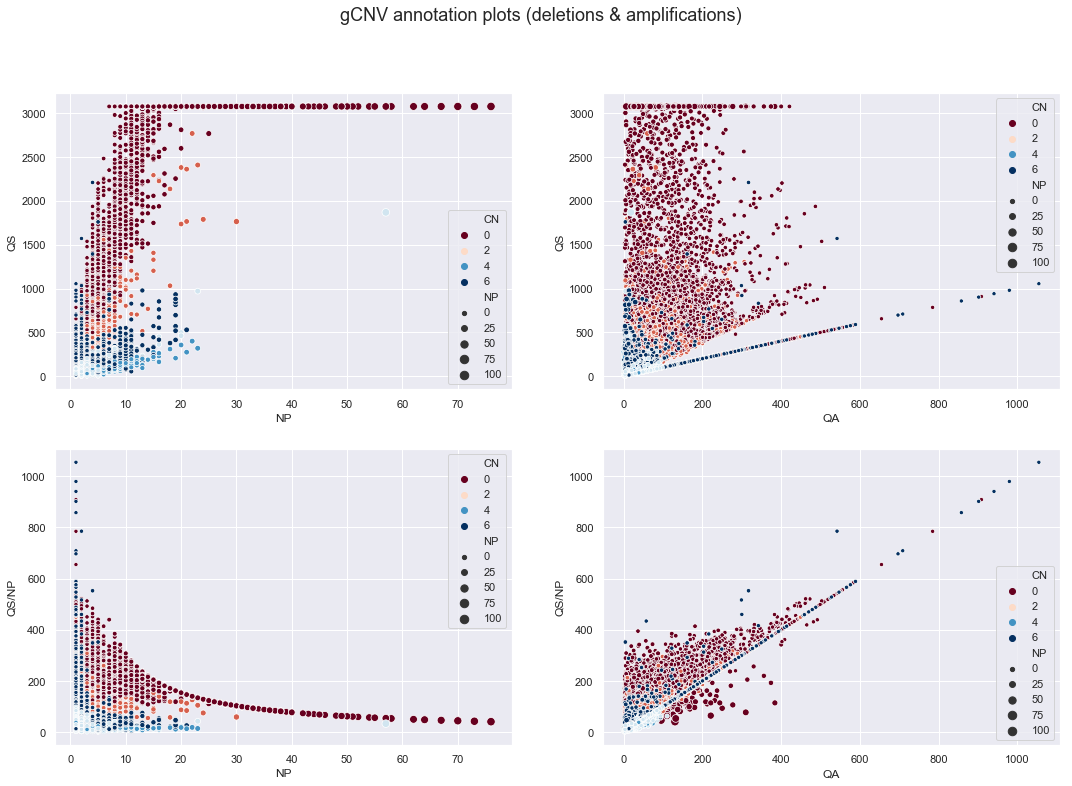This gCNV callset yields some beautiful plots. The clustering by CN is more prominant here.

• More segments are hitting the QS cap.
• Interestingly, the lower right plot shows a concentration of large segments below the diagnal line. These large segments have lower (QS/NP):QA ratios than smaller events.
```# Segment by event type and plot
fig, axs = plt.subplots(2,2, figsize=(18,12))

sns.scatterplot(x=b['NP'], y=b['QS']/b['NP'], hue=b['CN'], size=b['NP'], data=b, ax=axs[0,0], palette="mako")
sns.scatterplot(x=c['NP'], y=c['QS']/c['NP'], hue=c['CN'], size=c['NP'], data=c, ax=axs[0,1], palette="mako")
sns.scatterplot(x=b['QA'], y=b['QS']/b['NP'], hue=b['CN'], size=b['NP'], data=b, ax=axs[1,0], palette="mako")
sns.scatterplot(x=c['QA'], y=c['QS']/c['NP'], hue=c['CN'], size=c['NP'], data=c, ax=axs[1,1], palette="mako")

plt.suptitle('gCNV annotation plots', fontsize = 18)
axs[0,0].set_title('Deletions', fontsize=18)
axs[0,1].set_title('Amplifications', fontsize=18)
axs[0,0].set_ylabel('QS/NP', fontsize=12)
axs[0,1].set_ylabel('QS/NP', fontsize=12)
axs[1,0].set_ylabel('QS/NP', fontsize=12)
axs[1,1].set_ylabel('QS/NP', fontsize=12)

#axs[1,1].set_xlim(0,100)
#axs[1,1].set_ylim(0,100)
```
```Text(0, 0.5, 'QS/NP')
```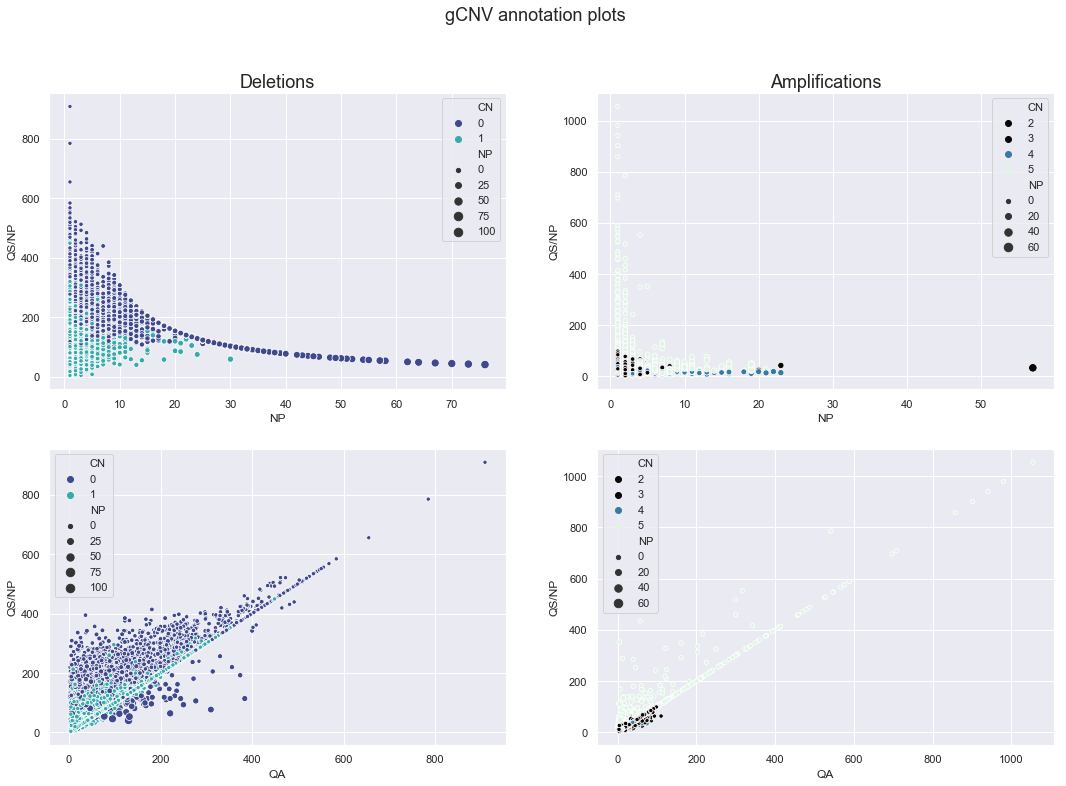# 3. Collect per-sample metrics and clustered heatmap of the metrics

Here, the analysis continues with the sensitive parameter gCNV results.

```binSize = 1000
sampleList = ["HG00096","HG00268","HG00419","HG00759","HG01051","HG01112","HG01500","HG01565",
"HG01583","HG01595","HG01879","HG02568","HG02922","HG03006","HG03052","HG03642",
"HG03742","NA18525","NA18939","NA19017","NA19625","NA19648","NA20502","NA20845"]
gcnvCohort = [];

for i in sampleList:
gcnvSample = pd.DataFrame(gcnvAllSensitive.loc[gcnvAllSensitive['sample'] == i])
b = gcnvSample.loc[gcnvSample['CN'] < 2, ['CN', 'NP', 'QA', 'QS']]
c = gcnvSample.loc[gcnvSample['CN'] > 2, ['CN', 'NP', 'QA', 'QS']]

gcnvSampleSummary = pd.DataFrame([np.count_nonzero(gcnvSample['CN'] == 0)], columns=['cn0'])
gcnvSampleSummary.insert(loc=0, column='sample', value=i)
gcnvSampleSummary['cn1'] = np.count_nonzero(gcnvSample['CN'] == 1)
gcnvSampleSummary['cn2'] = np.count_nonzero(gcnvSample['CN'] == 2)
gcnvSampleSummary['cn3'] = np.count_nonzero(gcnvSample['CN'] == 3)
gcnvSampleSummary['cn4'] = np.count_nonzero(gcnvSample['CN'] == 4)
gcnvSampleSummary['cn5'] = np.count_nonzero(gcnvSample['CN'] == 5)
gcnvSampleSummary['n-total'] = len(gcnvSample.index)
gcnvSampleSummary['n-dels'] = gcnvSampleSummary['cn0'] + gcnvSampleSummary['cn1']
gcnvSampleSummary['n-amps'] = gcnvSampleSummary['cn3'] + gcnvSampleSummary['cn4'] + gcnvSampleSummary['cn5']
gcnvSampleSummary['bases-dels'] = b['NP'].sum()*binSize
gcnvSampleSummary['bases-amps'] = c['NP'].sum()*binSize
gcnvSampleSummary['avg-del-size'] = round(gcnvSampleSummary['bases-dels'] / gcnvSampleSummary['n-dels']).astype(int)
gcnvSampleSummary['avg-amp-size'] = round(gcnvSampleSummary['bases-amps'] / gcnvSampleSummary['n-amps']).astype(int)
gcnvSampleSummary['atOrAbove1kb'] = len(gcnvSample[gcnvSample['NP'] >= 1])
gcnvSampleSummary['at1kb'] = len(gcnvSample[gcnvSample['NP'] == 1])
gcnvSampleSummary['fractionAt1kb'] = gcnvSampleSummary['at1kb']/gcnvSampleSummary['atOrAbove1kb']
gcnvSampleSummary['median-qa-dels'] = b['QA'].median()
gcnvSampleSummary['median-qa-amps'] = c['QA'].median()
gcnvSampleSummary['median-qs-dels'] = b['QS'].median()
gcnvSampleSummary['median-qs-amps'] = c['QS'].median()

gcnvCohort.append(gcnvSampleSummary[['sample','cn0', 'cn1', 'cn2', 'cn3', 'cn4', 'cn5', 'n-total', 'n-dels', 'n-amps',
'bases-dels', 'bases-amps', 'avg-del-size', 'avg-amp-size',
'at1kb', 'fractionAt1kb',
'median-qa-dels', 'median-qa-amps', 'median-qs-dels', 'median-qs-amps']])
gcnvCohort = pd.concat(gcnvCohort, axis=0, sort=False, ignore_index=True)
print ('Chromosome 20')
gcnvCohort
```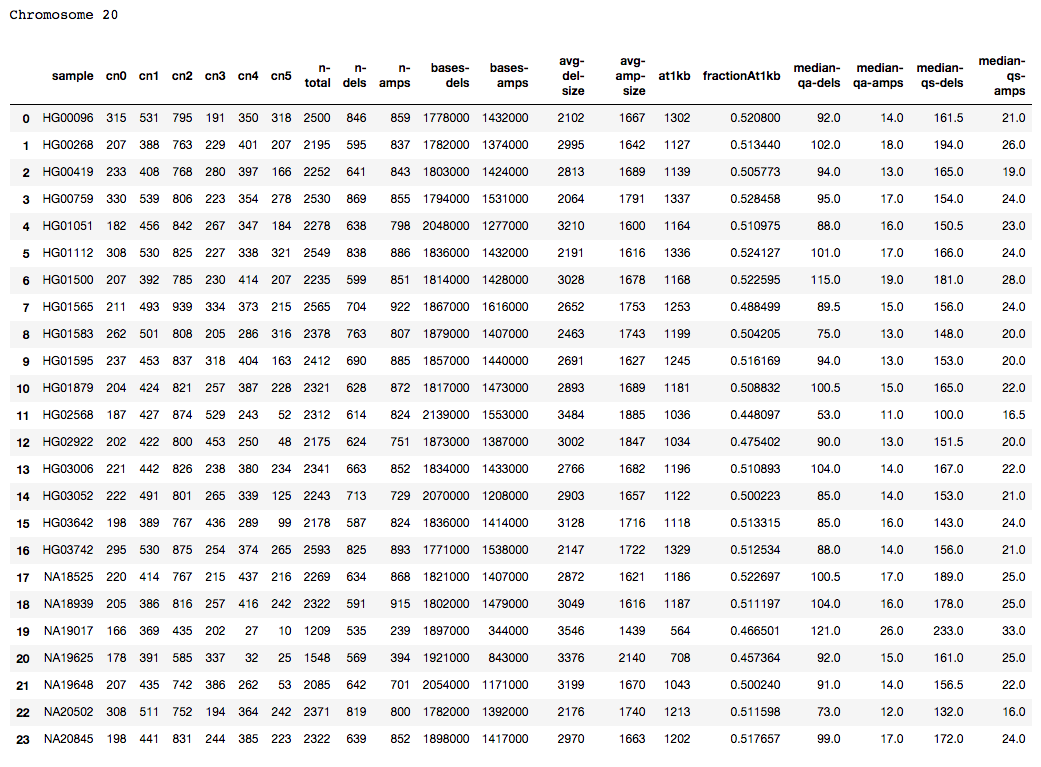Picking up trends by perusing the table by eye seems a tall order. Instead, visualize the data using a heatmap. Towards this, first normalize each data column.

```# Normalize data columns of interest by subtracting the mean and dividing by the standard deviation
gcnvCohortIndex = gcnvCohort
columnsToNormalize=['cn0', 'cn1', 'cn2', 'cn3', 'cn4', 'cn5', 'n-total', 'n-dels', 'n-amps',
'bases-dels', 'bases-amps', 'avg-del-size', 'avg-amp-size',
'at1kb', 'fractionAt1kb',
'median-qa-dels', 'median-qa-amps', 'median-qs-dels', 'median-qs-amps']
gcnvCohortNorm=(gcnvCohortIndex[columnsToNormalize]-gcnvCohortIndex[columnsToNormalize].mean())/gcnvCohortIndex[columnsToNormalize].std()
gcnvCohortNorm.insert(loc=0, column='sample', value=gcnvCohort['sample'])
gcnvCohortNorm=gcnvCohortNorm.set_index('sample')
```

A heatmap allows visualization of the metrics. A clustered heatmap further enables grouping the samples, as well as the metrics, by those that correlate.

```# Generate clustered heatmap
sns.clustermap(gcnvCohortNorm,cmap="mako")
```
```<seaborn.matrix.ClusterGrid at 0x14be04ef0>
```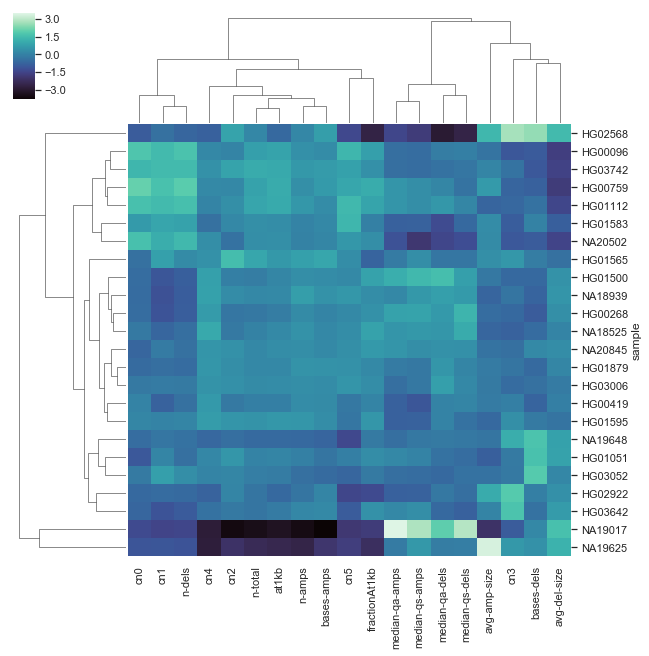At glance, three samples stand out from the rest. Instead of sample names, we can label the rows by population to see whether the larger groupings correlate.

```# Replace sample names with 1000 Genomes Project super population grouping
# See <http://www.internationalgenome.org/category/population/> for designations
gcnvSuper = gcnvCohortNorm.merge(superGroup[['entity:participant_id','super_population']], how='left', left_index=True, right_on='entity:participant_id')
gcnvSuper=gcnvSuper.set_index('super_population')
```
```# Redraw clustered heatmap with super population labels
sns.clustermap(gcnvSuper.iloc[:,0:-1],cmap="mako")
```
```<seaborn.matrix.ClusterGrid at 0x14c0ca8d0>
```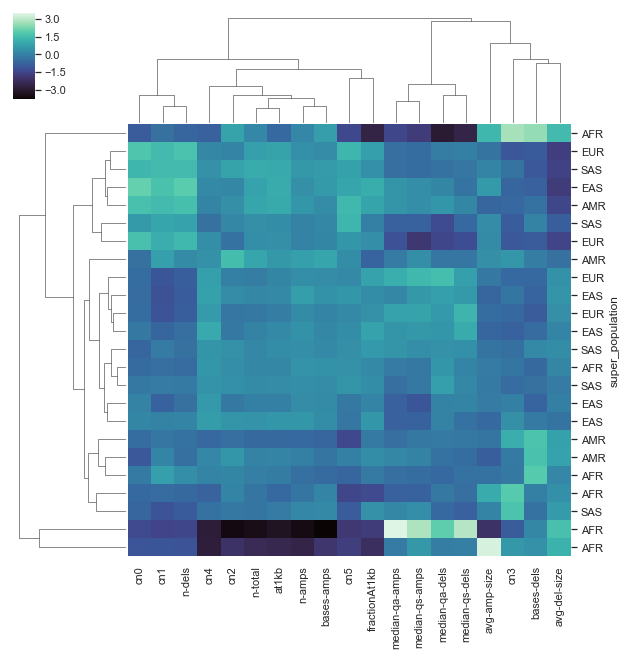Post edited by shlee on